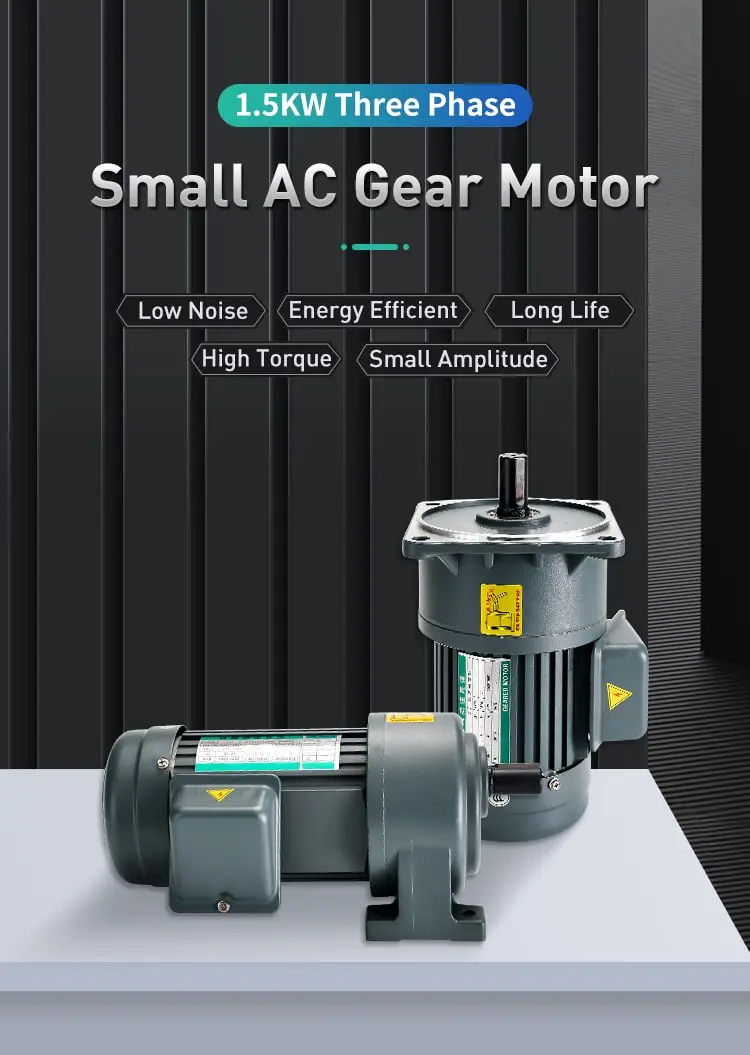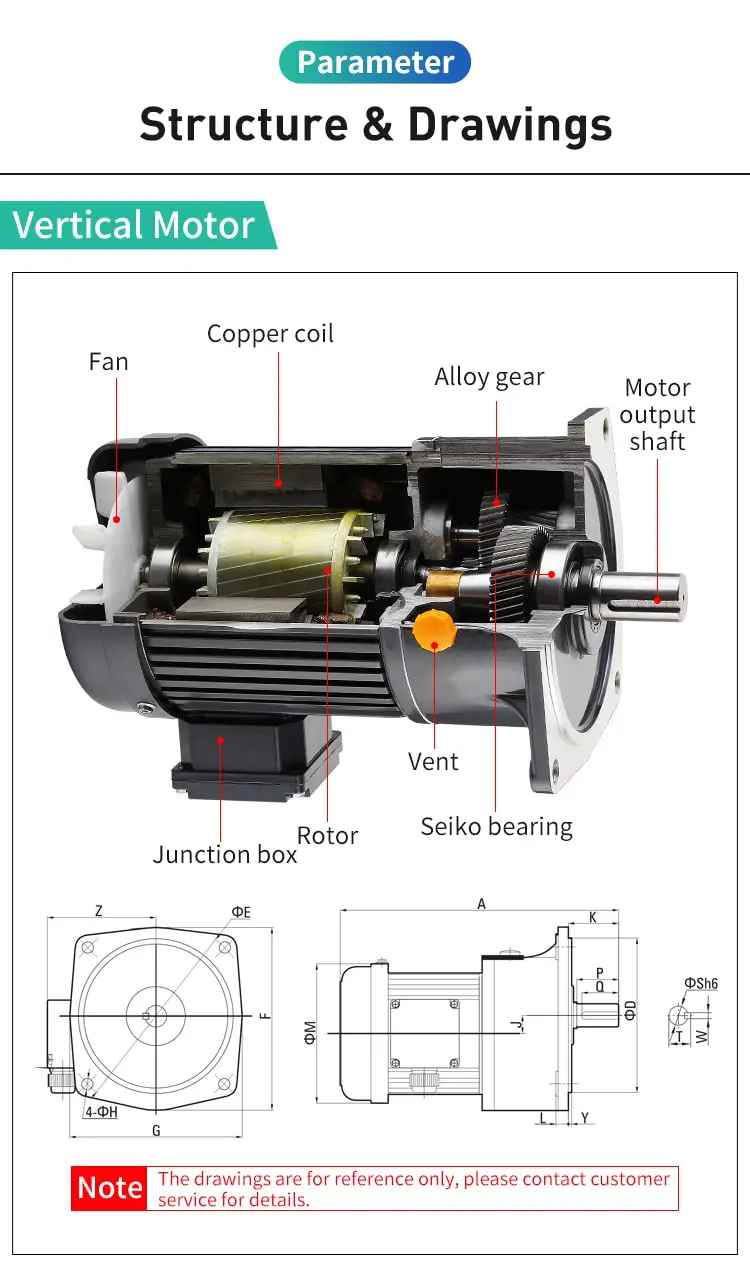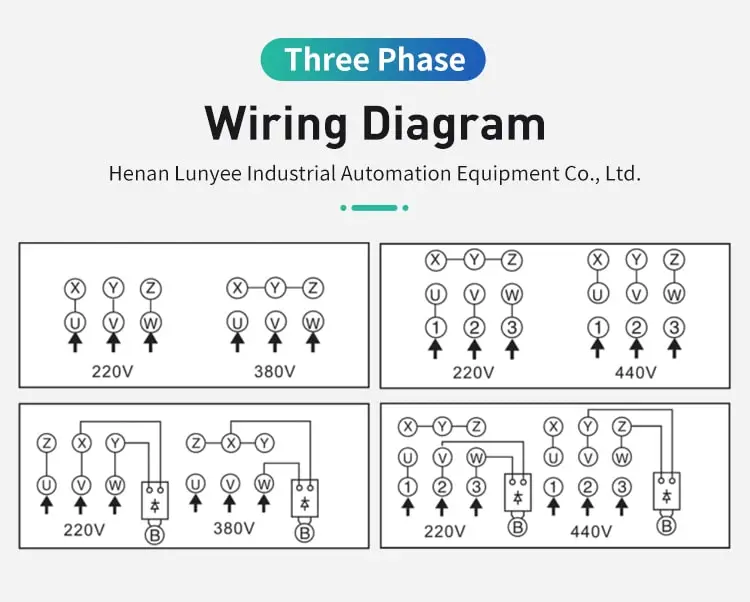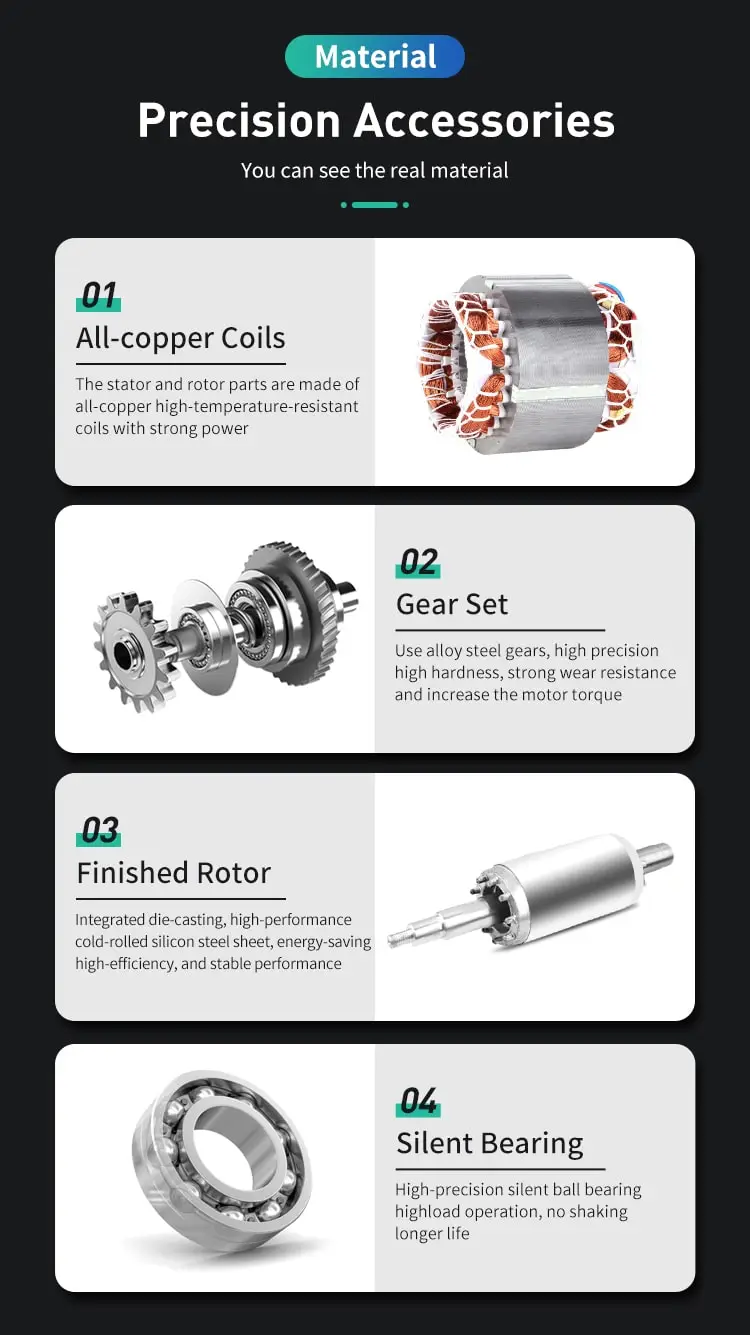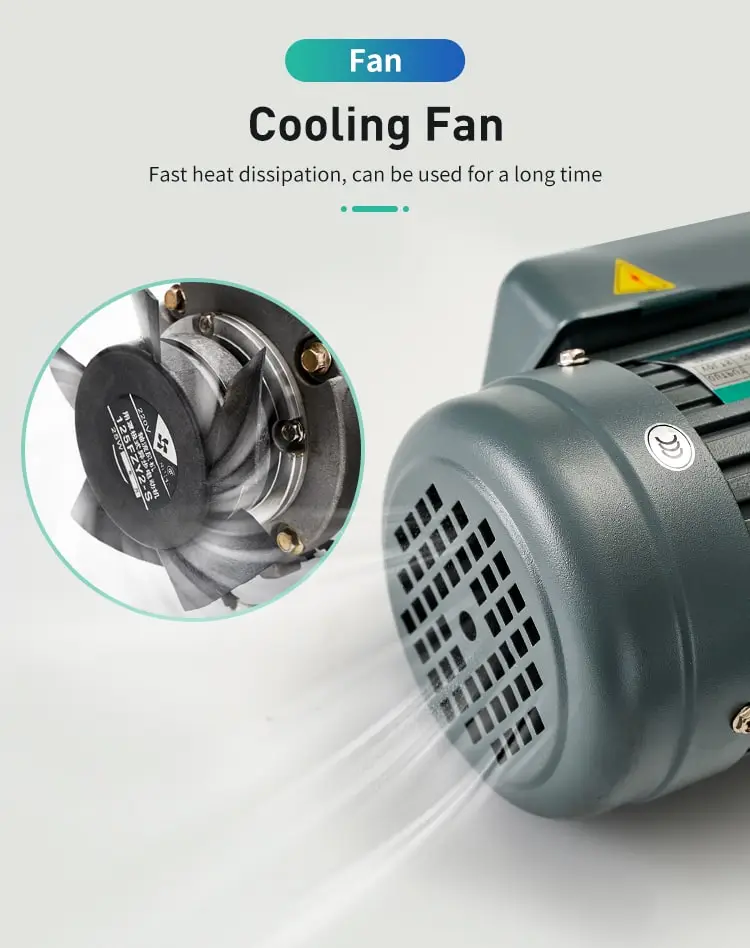By Jasmine 2023-02-17 15:57:13

#### How to calculate efficiency of induction motor?

To calculate a motor efficiency, multiply the horsepower by the load ratio, divide by the input power, and finally multiply by 74.57

The efficiency of an induction motor can be calculated using the following formula:

Efficiency = (Output Power / Input Power) x 100%

To calculate the efficiency of an induction motor, you will need to measure the input power and the output power of the motor. The input power of the motor can be measured by using a wattmeter or a power meter, and the output power of the motor can be measured by using a torque meter or a tachometer.

Once you have measured the input and output power of the induction motor, you can use the above formula to calculate the efficiency of the motor. For example, if the input power of the motor is 1000 watts and the output power of the motor is 800 watts, then the efficiency of the motor would be calculated as follows:

Efficiency = (800 watts / 1000 watts) x 100% = 80%

In this example, the efficiency of the induction motor is 80%, which means that 80% of the input power is converted into useful output power, and the remaining 20% is lost as heat or other forms of energy.

In summary, the efficiency of an induction motor can be calculated using the formula: Efficiency = (Output Power / Input Power) x 100%. To calculate the efficiency of an induction motor, you will need to measure the input and output power of the motor, and then use the above formula to calculate the efficiency of the motor.